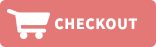Home » Shelf Strips-Add 25% to listed price. » 1.22" x .010", PVC SHELF STRIPS (CLEAR)Product ID: ShelfStripST1208U100
Shelf Strips

The shelf strip measures 1.22" x 47.75" x .010".  Prices decrease as volume increases.  Store will automatically calculate discounts.100 strips per bundle, for quantities of 10 bundles or more see the 1,000 count section.

Quantity pricing per 100:
1= \$29.98
2= \$21.81
3= \$18.91
5= \$16.74
Price: \$29.98Product ID: ShelfStripST1208U1000
Shelf Strips

The shelf strip measures 1.22" x 47.75" x .010".  Prices decrease as volume increases.  Store will automatically calculate discounts.1,000 strips per case, for quantities of 900 strips or less, please see the 100 count section.

Quantity Pricing per 1,000:
1= \$141.60
2= \$136.30
3= \$127.30
5= \$117.60
10= \$109.40
25= \$100.20
50= \$94.60
100= \$88.30
Price: \$141.60Product ID: ShelfStripST2127100
Shelf Strips

The shelf strip measures 1.22" x 24" x .010".  Prices decrease as volume increases.  Store will automatically calculate discounts. 100 strips per bundle, for quantities of 10 bundles or more see the 1,000 count section.

Quantity Pricing per 100:
1= \$21.82
2= \$15.63
3= \$14.22
5= \$11.93
Price: \$21.82Product ID: ShelfStripST21271000
Shelf Strips

The shelf strip measures 1.22" x 24" x .010".  Prices decrease as volume increases.  Store will automatically calculate discounts. 1,000 strips per case, for quantities of 900 strips or less, please see the 100 count section.

Quantity Pricing per 1,000:
1= \$101.02
2= \$98.40
5= \$85.70
10= \$79.80
25= \$73.30
50= \$68.90
100= \$65.50
Price: \$101.02